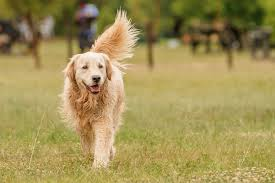# Image recognition with ResNet50v2 in C#

The sample walks through how to run a pretrained ResNet50 v2 ONNX model using the Onnx Runtime C# API.

The source code for this sample is available here.

## Prerequisites

To run this sample, you’ll need the following things:

1. Install .NET Core 3.1 or higher for you OS (Mac, Windows or Linux).
3. Download this picture of a dog to test the model. You can also use any image you like.

## Getting Started

Now we have everything set up, we can start adding code to run the model on the image. We’ll do this in the main method of the program for simplicity.

Firstly, let’s read the path to the model and path to the image we want to test in through program arguments:

``````string modelFilePath = args;
string imageFilePath = args;
``````

Next, we will read the image in using the cross-platform image library ImageSharp:

``````using Image<Rgb24> image = Image.Load<Rgb24>(imageFilePath, out IImageFormat format);
``````

Note, we’re specifically reading the `Rgb24` type so we can efficiently preprocess the image in a later step.

### Resize image

Next, we will resize the image to the appropriate size that the model is expecting; 224 pixels by 224 pixels:

``````using Stream imageStream = new MemoryStream();
image.Mutate(x =>
{
x.Resize(new ResizeOptions
{
Size = new Size(224, 224),
Mode = ResizeMode.Crop
});
});
image.Save(imageStream, format);
``````

Note, we’re doing a centered crop resize to preserve aspect ratio.

### Preprocess image

Next, we will preprocess the image according to the requirements of the model:

``````Tensor<float> input = new DenseTensor<float>(new[] { 1, 3, 224, 224 });
var mean = new[] { 0.485f, 0.456f, 0.406f };
var stddev = new[] { 0.229f, 0.224f, 0.225f };
for (int y = 0; y < image.Height; y++)
{
Span<Rgb24> pixelSpan = image.GetPixelRowSpan(y);
for (int x = 0; x < image.Width; x++)
{
input[0, 0, y, x] = ((pixelSpan[x].R / 255f) - mean) / stddev;
input[0, 1, y, x] = ((pixelSpan[x].G / 255f) - mean) / stddev;
input[0, 2, y, x] = ((pixelSpan[x].B / 255f) - mean) / stddev;
}
}
``````

Here, we’re creating a Tensor of the required size `(batch-size, channels, height, width)`, accessing the pixel values, preprocessing them and finally assigning them to the tensor at the appropriate indicies.

### Setup inputs

Next, we will create the inputs to the model:

``````var inputs = new List<NamedOnnxValue>
{
NamedOnnxValue.CreateFromTensor("data", input)
};
``````

To check the input node names for an ONNX model, you can use Netron to visualise the model and see input/output names. In this case, this model has `data` as the input node name.

### Run inference

Next, we will create an inference session and run the input through it:

``````using var session = new InferenceSession(modelFilePath);
``````

### Postprocess output

Next, we will need to postprocess the output to get the softmax vector, as this is not handled by the model itself:

``````IEnumerable<float> output = results.First().AsEnumerable<float>();
float sum = output.Sum(x => (float)Math.Exp(x));
IEnumerable<float> softmax = output.Select(x => (float)Math.Exp(x) / sum);
``````

Other models may apply a Softmax node before the output, in which case you won’t need this step. Again, you can use Netron to see the model outputs.

### Extract top 10

Next, we will extract the top 10 class predictions:

``````IEnumerable<Prediction> top10 = softmax.Select((x, i) => new Prediction { Label = LabelMap.Labels[i], Confidence = x })
.OrderByDescending(x => x.Confidence)
.Take(10);
``````

Next, we will print the top 10 results to the console:

``````Console.WriteLine("Top 10 predictions for ResNet50 v2...");
Console.WriteLine("--------------------------------------------------------------");
foreach (var t in top10)
{
Console.WriteLine(\$"Label: {t.Label}, Confidence: {t.Confidence}");
}
``````

## Running the program

Now the program is created, we can run it will the following command:

``````dotnet run [path-to-model] [path-to-image]
``````

e.g.

``````dotnet run ~/Downloads/resnet50-v2-7.onnx ~/Downloads/dog.jpeg
``````

Running this on the following image:We get the following output:

``````Top 10 predictions for ResNet50 v2...
--------------------------------------------------------------
Label: Golden Retriever, Confidence: 0.9212826
Label: Kuvasz, Confidence: 0.026514154
Label: Clumber Spaniel, Confidence: 0.012455719